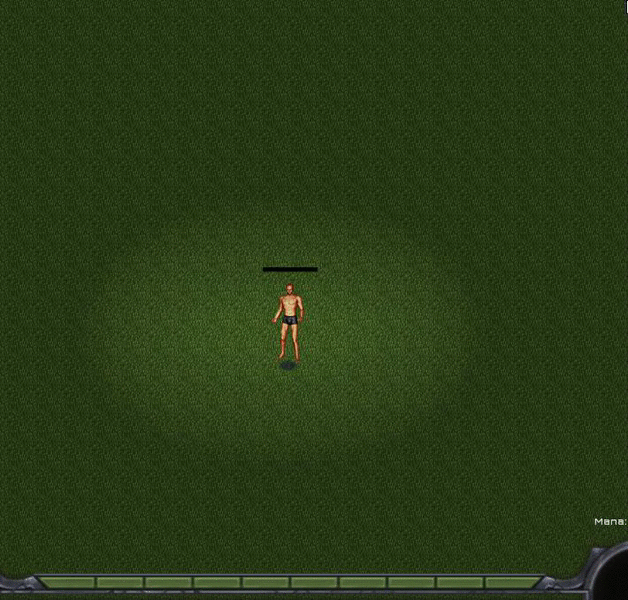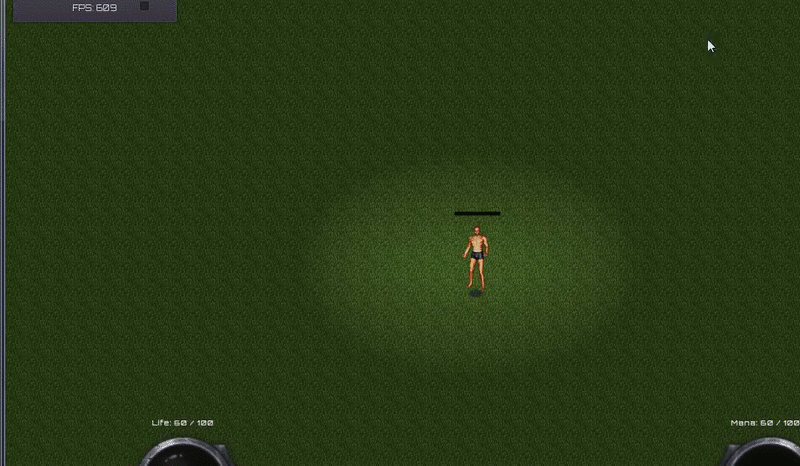Alrighty, so this is what I get for failing Math so bad at school! Forgive me in advance...

Anyways.

My character basically clicks anywhere on the map, and he gets to shoot a fireball:

Here is the code for that:

``````    for number_of_projectiles in range(3):
var temp_skill = scene.instance()
var angle = gg.players.get_global_mouse_pos().angle_to_point(gg.players.get_pos())

var mpos = gg.players.get_global_mouse_pos()
var ang = gg.players.get_angle_to(mpos)
temp_skill.set_pos(gg.players.get_pos())
temp_skill.add_collision_exception_with(gg.players) # Cannot collide with self

for x in gg.Live_Skills:
temp_skill.add_collision_exception_with(gg.Live_Skills[x]) # Cannot collide with these same bodies

var X  = 250 * sin(angle);
var Y  = 250 * cos(angle);
temp_skill.newMove = Vector2(X, Y)

gg.Live_Skills[temp_skill.get_name()] = temp_skill
``````

And for my Fireball.gd:

``````func _fixed_process(delta):
if (hit):
return
move(newMove*delta)
if (is_colliding()):
get_node("anim").play("explode")
hit = true
``````

So, for this example there is 3 projectiles (`number_of_projectiles`)

I need to find the Math algorithm here to separate them out evenly, for example. If I am shooting `3` projectiles, it shows them overlapping:I need to play with `number_of_projectiles` and use them with the `sin` and `cos` methods so I can evenly separate them into a "projectile" wave. This is where I'm stuck because I just am not sure how to do it.

I've tried `sin(angle) * number_of_projectiles` but, with unexpected results (the fireballs all go at different speeds and in 1 straight line).

in Engine
edited

+1 vote

If I understood correctly, you need to avoid the overlapping of the projectile.

I am on mobile now, so I will post what to do.

1. You need to have a timer variable and set its value as 1.0.
2. Then in every update, reduce it by delta.
3. Inside the projectile create method, create the projectile only if the timer <=0 and reset the timer back to 1.0

So you will get a 1.0 second delay between the fireballs.

by (750 points)
selected

@vlnod, thanks. I'll try something like that now. The problem is, the projectiles cannot have a delay though, but be spread out in a wave. Apologizes for my poor wording, take a look here: https://i.gyazo.com/d40f860d362763bad6d5c6eccd41b4fd.png

OK. Now I understood.

Again I am on mobile and I will tell you what to do.

You already found that your angle variable is directed towards the firing direction.
So you need multiple angles for each projectile.

For eg, if you have 5 projectiles in a wave, then you need,
angle-2x, angle-x, angle, angle+x, angle+2x
where x is a value you need to figure out. If your angle is in radians it will be small. This x represents the separation between each projectile.

Put the above five angles for each projectile and it should work.

Divide numofprojectiles by 2 and round it. Then you will get a number. You will need to find that much angles on right and left side of your main angle.

For eg, if number of projectiles is 5, round(5/2)=2
So you need to find 2 angles to the positive side and 2 angles to the negative side.
Hope you understood

Awesome @vlnod. I added something like this:

`angle -= 0.1 * i`

`i` = number of projectilesNow, problem is, it's not evenly spaced. the wave goes up higher than the mouse position. Hmmmm

Thanks for your help so far, I think I a understanding it now

Happy to know you are getting results.

You can check the distance between mouse and the target position.
At the same time check each projectile current position and the spawn position. After covering the mouse distance remove the projectile.

To make the spacing between each waves consistent, you can set a timer and add a delay as I told earlier to each wave.

Old queston, but for the ones who also had the same problem.

You can create an array of angles based on the number of projectiles, that way the angle will add one side at a time, not just for one side, and the first bullet will be in the middle where your mouse is pointing.

``````var projectiles_angles = 
for i in range(1, projectiles * 2):
var num = float(i) / 10
projectiles_angles.append(num)
projectiles_angles.append(num * -1)
``````

This will produce `[0, 1, -1, 2, -2, 3, -3, 4, -4]` and so on.

``````angle += projectiles_angles[i]
``````

Full code:

``````func fire_projectile(delta):
var projectiles_angles = 
for i in range(1, projectiles * 2):
var num = float(i) / 10
projectiles_angles.append(num)
projectiles_angles.append(num * -1)

for i in range(projectiles):
var bullet_instance = bullet.instance()
var angle = get_angle_to(get_global_mouse_position())
angle += projectiles_angles[i]
bullet_instance.position = bullet_point.global_position
bullet_instance.rotation = angle